Anúncio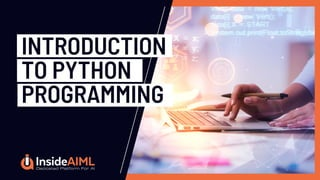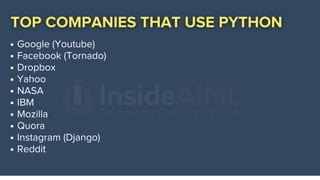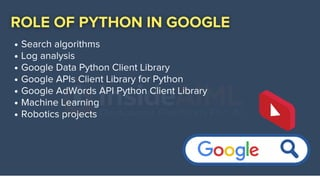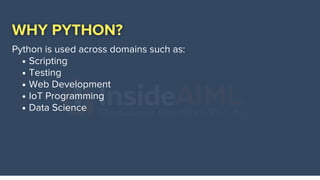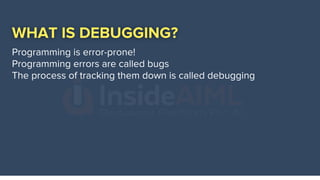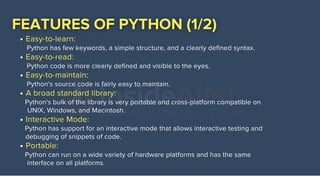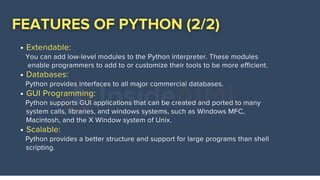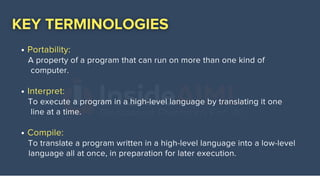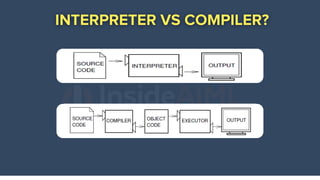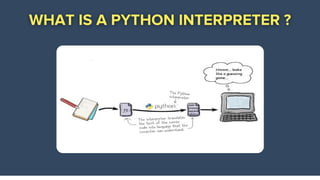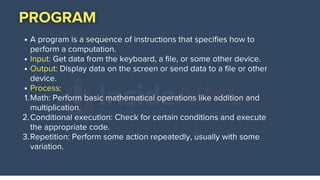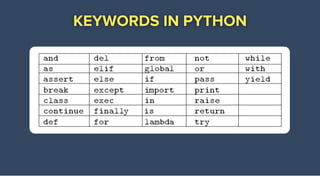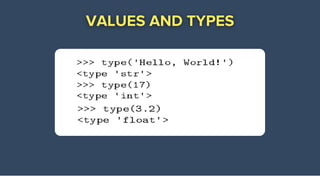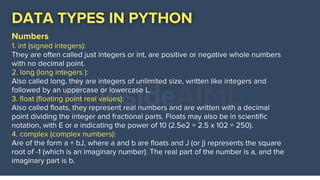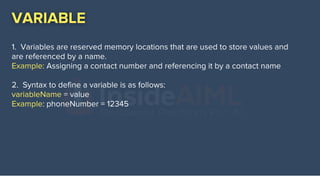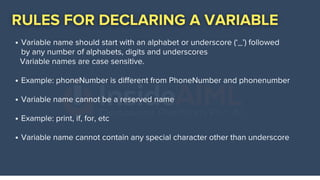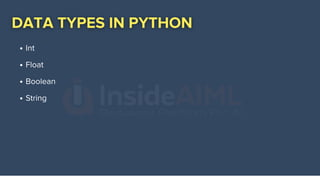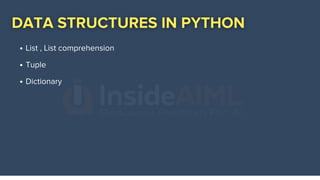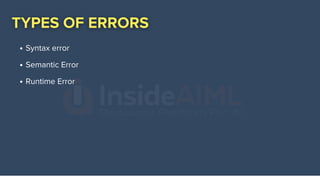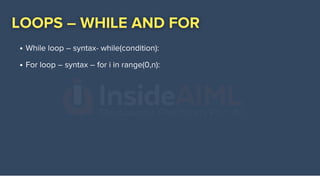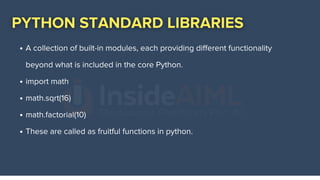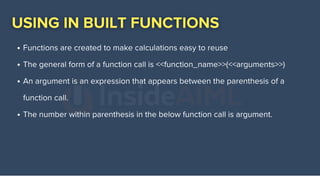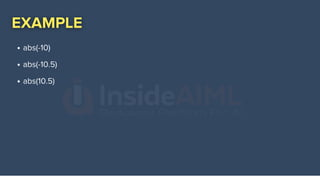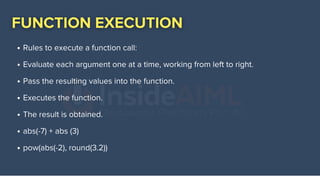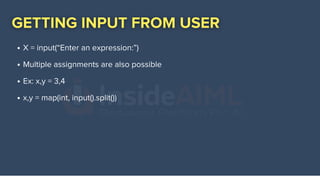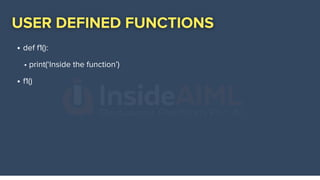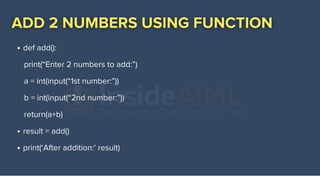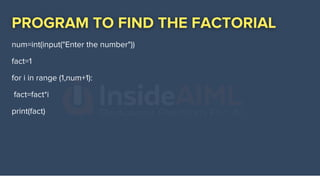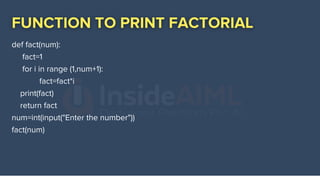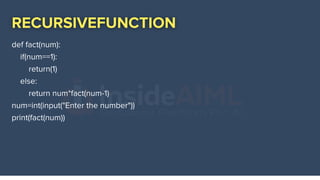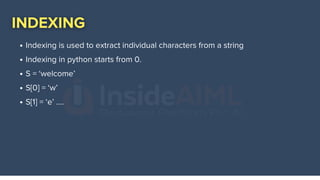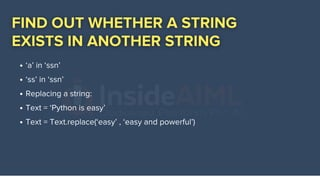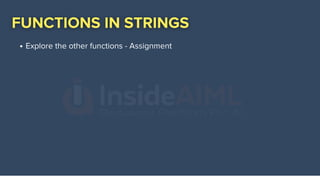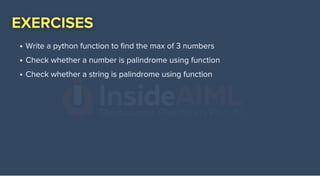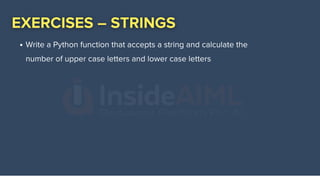1 de 42
Anúncio

### Introduction to Python Programming | InsideAIML

1. INTRODUCTION TO PYTHON PROGRAMMING
3. Search algorithms Log analysis Google Data Python Client Library Google APIs Client Library for Python Google AdWords API Python Client Library Machine Learning Robotics projects
4. NAME IS BASED ON A BBC COMEDY SERIES FROM THE 1970S Monty Python's Flying Circus Guido van Rossum December 1989
5. Scripting Testing Web Development IoT Programming Data Science Python is used across domains such as:
6. Programming is error-prone! Programming errors are called bugs The process of tracking them down is called debugging
7. Easy-to-learn: Easy-to-read: Easy-to-maintain: A broad standard library: Interactive Mode: Portable: Python has few keywords, a simple structure, and a clearly defined syntax. Python code is more clearly defined and visible to the eyes. Python's source code is fairly easy to maintain. Python's bulk of the library is very portable and cross-platform compatible on ....... UNIX, Windows, and Macintosh. Python has support for an interactive mode that allows interactive testing and .......debugging of snippets of code. Python can run on a wide variety of hardware platforms and has the same ........interface on all platforms.
8. Extendable: Databases: GUI Programming: Scalable: You can add low-level modules to the Python interpreter. These modules ........enable programmers to add to or customize their tools to be more efficient. Python provides interfaces to all major commercial databases. Python supports GUI applications that can be created and ported to many .......system calls, libraries, and windows systems, such as Windows MFC, .......Macintosh, and the X Window system of Unix. Python provides a better structure and support for large programs than shell ........scripting.
9. Portability: Interpret: Compile: A property of a program that can run on more than one kind of ........computer. To execute a program in a high-level language by translating it one ........line at a time. To translate a program written in a high-level language into a low-level ........language all at once, in preparation for later execution.
10. A program is a sequence of instructions that specifies how to perform a computation. Input: Get data from the keyboard, a file, or some other device. Output: Display data on the screen or send data to a file or other device. Process: Math: Perform basic mathematical operations like addition and multiplication. Conditional execution: Check for certain conditions and execute the appropriate code. Repetition: Perform some action repeatedly, usually with some variation. 1. 2. 3.
11. HTTPS://REPO.ANACONDA.COM/ARCHIVE/
12. Numbers 1. int (signed integers): They are often called just integers or int, are positive or negative whole numbers with no decimal point. 2. long (long integers ): Also called long, they are integers of unlimited size, written like integers and followed by an uppercase or lowercase L. 3. float (floating point real values): Also called floats, they represent real numbers and are written with a decimal point dividing the integer and fractional parts. Floats may also be in scientific notation, with E or e indicating the power of 10 (2.5e2 = 2.5 x 102 = 250). 4. complex (complex numbers): Are of the form a + bJ, where a and b are floats and J (or j) represents the square root of -1 (which is an imaginary number). The real part of the number is a, and the imaginary part is b.
13. 1. Variables are reserved memory locations that are used to store values and are referenced by a name. Example: Assigning a contact number and referencing it by a contact name 2. Syntax to define a variable is as follows: variableName = value Example: phoneNumber = 12345
14. Variable name should start with an alphabet or underscore (‘_’) followed by any number of alphabets, digits and underscores Example: phoneNumber is different from PhoneNumber and phonenumber Variable name cannot be a reserved name Example: print, if, for, etc Variable name cannot contain any special character other than underscore Variable names are case sensitive.
15. Int Float Boolean String
16. List , List comprehension Tuple Dictionary
17. Syntax error Semantic Error Runtime Error
18. While loop – syntax- while(condition): For loop – syntax – for i in range(0,n):
19. A collection of built-in modules, each providing different functionality beyond what is included in the core Python. import math math.sqrt(16) math.factorial(10) These are called as fruitful functions in python.
20. Functions are created to make calculations easy to reuse The general form of a function call is <<function_name>>(<<arguments>>) An argument is an expression that appears between the parenthesis of a function call. The number within parenthesis in the below function call is argument.
21. abs(-10) abs(-10.5) abs(10.5)
22. Rules to execute a function call: Evaluate each argument one at a time, working from left to right. Pass the resulting values into the function. Executes the function. The result is obtained. abs(-7) + abs (3) pow(abs(-2), round(3.2))
23. X = input(“Enter an expression:”) Multiple assignments are also possible Ex: x,y = 3,4 x,y = map(int, input().split())
24. def f1(): f1() • print(‘Inside the function’)
25. def f1(number): F1(5) •print(number)
26. def add(): result = add() print(‘After addition:’ result) print(“Enter 2 numbers to add:”) a = int(input(“1st number:”)) b = int(input(“2nd number:”)) return(a+b)
27. num=int(input("Enter the number")) fact=1 for i in range (1,num+1): fact=fact*i print(fact)
28. fact(num) def fact(num): fact=1 for i in range (1,num+1): fact=fact*i print(fact) return fact num=int(input("Enter the number"))
29. print(fact(num)) def fact(num): if(num==1): return(1) else: return num*fact(num-1) num=int(input("Enter the number"))
30. Strings are a collection of characters. They are enclosed in single or double-quotes. Eg: ‘Climate is pleasant’ ‘1234’ ‘#@\$’
31. Operators overloading are available in default. 3*’a’ = ‘aaa’ ‘a’+’a’ = ‘aa’ ‘a’ + 3 will give synatax error (you cannot add a string and a number) ‘a’ + ‘3’ = ‘a3’ ‘a’*’a’ will give a syntax error.
32. Indexing is used to extract individual characters from a string Indexing in python starts from 0. S = ‘welcome’ S = ‘w’ S = ‘e’ ….
33. Slicing is taking subsets from a string. Lower limit is inclusive where as Upper limit is exclusive. S=‘welcome’ S[0:2] ?? S[0:] ?? S[:3] ?? S[:] ??? S[0:4]??
34. ‘a’ in ‘ssn’ ‘ss’ in ‘ssn’ Replacing a string: Text = ‘Python is easy’ Text = Text.replace(‘easy’ , ‘easy and powerful’)
35. Explore the other functions - Assignment
36. Write a python function to find the max of 3 numbers Check whether a number is palindrome using function Check whether a string is palindrome using function
37. Write a Python function that accepts a string and calculate the number of upper case letters and lower case letters
Anúncio